ramo

•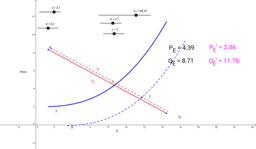How Shifts in Supply and Demand curves affect P and Q

Activity

ramo

•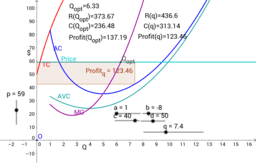Maximizing Profit by Adjusting Quantity: perfect competition

Activity

ramo

•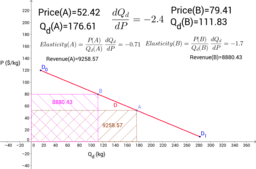Total Revenue and Price Elasticity of Demand

Activity

ramo

•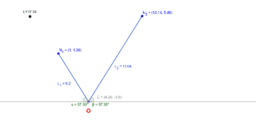mass freely dangling from a string supported by two pegs II

Activity

ramo

•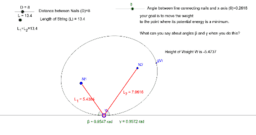mass freely dangling from a string supported by two pegs

Activity

ramo

•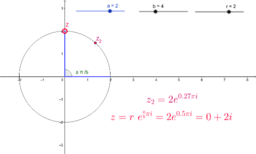Tool to aid teachers wishing to intoduce Euler's formula to

Activity

ramo

•Law of Sines (proof using vectors)

Activity

ramo

•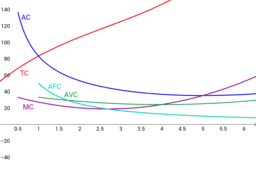Production Costs (fixed and variable) Average and Marginal

Activity

ramo

•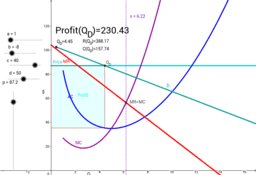How does a monopolist decide upon price?

Activity

ramo

•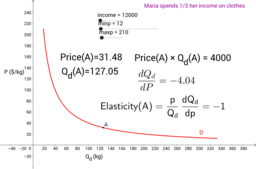Price Elasticity of Demand - Hypothetical Maria

Activity

ramo

•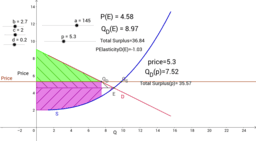Efficient Markets maximize Total Surplus

Activity

ramo

•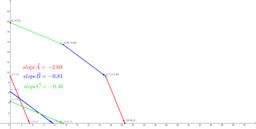Production Possibilites Frontiers

Activity

ramo

•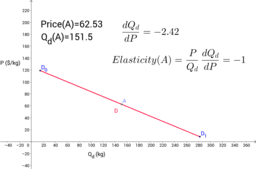How Price Elasticity of Demand varies along a Demand Curve

Activity

ramo

•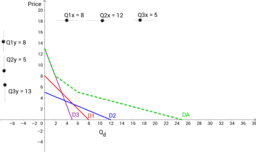Market Demand Curve construction by horizontal addition

Activity

ramo

•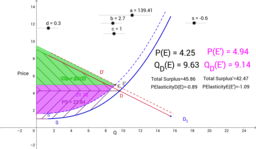Price Elasticity, and Supply and Demand Curves

Activity

ramo

•Income Elasticity of Demand

Activity

ramo

•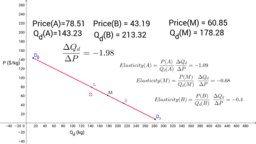Price Elasticity of Demand - point to point vs. midpoint

Activity

ramo

•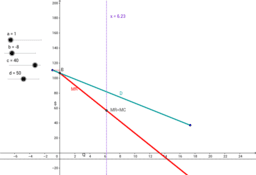A Monopolist's MR curve when Demand is linear

Activity

ramo

•ramo's Microeconomics teaching aids

Book

ramo

•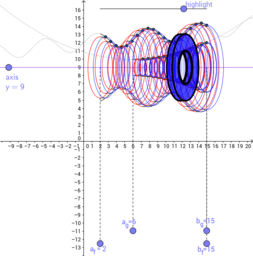Book

ramo